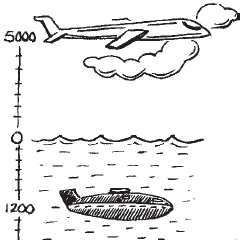# A plane is flying at the height of 5000 m above the sea level. At a particular point, it is exactly above a submarine floating 1200 m below the sea level. What is the vertical distance between them?Solution

From the given details we get to know that

The plane is flying at the height = 5000 m

Depth of Submarine = -1200 m

Distance between plane and submarine = 5000 m − (−1200) m

= 5000 m + 1200 m = 6200 m

Distance between plane and submarine = 6200 m(0)(0)